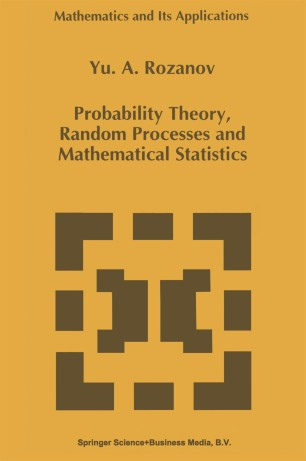Advertisement

# Probability Theory, Random Processes and Mathematical Statistics

• Yu. A. RozanovBook

Part of the Mathematics and Its Applications book series (MAIA, volume 344)

## Table of contents

1. Front Matter
Pages i-xi
2. Yu. A. Rozanov
Pages 1-90
3. Yu. A. Rozanov
Pages 91-129
4. Yu. A. Rozanov
Pages 131-170
5. Yu. A. Rozanov
Pages 171-200
6. Yu. A. Rozanov
Pages 201-252
7. Back Matter
Pages 253-259

## About this book

### Introduction

Probability Theory, Theory of Random Processes and Mathematical Statistics are important areas of modern mathematics and its applications. They develop rigorous models for a proper treatment for various 'random' phenomena which we encounter in the real world. They provide us with numerous tools for an analysis, prediction and, ultimately, control of random phenomena. Statistics itself helps with choice of a proper mathematical model (e.g., by estimation of unknown parameters) on the basis of statistical data collected by observations. This volume is intended to be a concise textbook for a graduate level course, with carefully selected topics representing the most important areas of modern Probability, Random Processes and Statistics. The first part (Ch. 1-3) can serve as a self-contained, elementary introduction to Probability, Random Processes and Statistics. It contains a number of relatively sim­ ple and typical examples of random phenomena which allow a natural introduction of general structures and methods. Only knowledge of elements of real/complex analysis, linear algebra and ordinary differential equations is required here. The second part (Ch. 4-6) provides a foundation of Stochastic Analysis, gives information on basic models of random processes and tools to study them. Here a familiarity with elements of functional analysis is necessary. Our intention to make this course fast-moving made it necessary to present important material in a form of examples.

### Keywords

Algebra Probability theory differential equation functional analysis linear algebra mathematical statistics model ordinary differential equation statistics

#### Authors and affiliations

• Yu. A. Rozanov
• 1
1. 1.Steklov Mathematical InstituteMoscowRussia

### Bibliographic information

• DOI https://doi.org/10.1007/978-94-011-0449-4
• Copyright Information Kluwer Academic Publishers 1995
• Publisher Name Springer, Dordrecht
• eBook Packages
• Print ISBN 978-94-010-4201-7
• Online ISBN 978-94-011-0449-4
• Buy this book on publisher's site